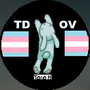1. Forum
2. >
3. Topic: Duolingo
4. >
5. What is the threshold for eac…# What is the threshold for each level up? How much exp for level 25?

EDIT: I found them and they are as follows: 2=60 3=120 4=200 5=300 6=350 7=750 8=1125 9=1650 10=2250 11=3000 12=3900 13=4900 14=6000 15=7500 16=9000 17=10500 18=12000 19=13500 20=15000 21=17000 22=19000 23=22500 24=26000 **25=30000

September 22, 2017Hi! Here is the Duolingo Wiki's chart for how many XP between each level.

To get to level 25, you need to earn 30,000 XP :)•1148

The xp needed to level up increase as your level goes up. You need 60 xp to level up to level 2 and 4,000 to go from level 24 to level 25. You need 30,000 points total to reach level 25.